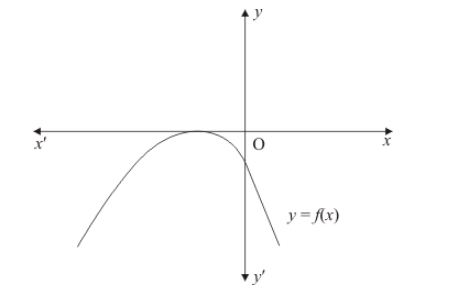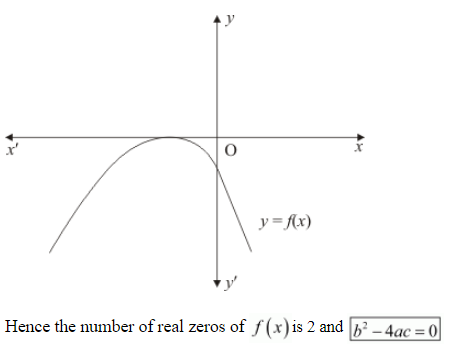# The graph of the polynomial f(x) = ax2 + bx + c is as shown`
Question:

The graph of the polynomial $f(x)=a x^{2}+b x+c$ is as shown in Fig. 2.20. Write the value of $b^{2}-4 a c$ and the number of real zeros of $f(x)$.Solution:

The graph of the polynomial $f(x)=a x^{2}+b x+c$ or the curve touches $x$-axis at point $\left(\frac{-b}{2 a}, 0\right)$. The $x$-coordinate of this point gives two equal zeros of the polynomial and $b^{2}-4 a c=0$.